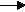gcsescience.com                                       27                                       gcsescience.com

Moles

Masses or Volumes from Electrolysis.

Example 4.

The electrolysis of molten aluminium oxide - Al2O3
produces aluminium at the cathode and oxygen at the anode.
See the extraction of aluminium for more detail.

A current of 10 amps is allowed to flow
through molten aluminium oxide for 5 hours.
What mass of aluminium is deposited at the cathode?

Method.
1) Write the half equation for the electrolysis.
Al3+  +    3e-Al
3 moles of electrons (3 faradays)
are required to deposit 1 mole of aluminium.

2) Find how many faradays have passed through
the aluminium oxide in 5 hours.

Q = I x t

5 hours contains (5 x 60 x 60) seconds
= 18,000 seconds.

Q = 10 x 18,000
= 180,000 coulombs.

180,000 coulombs = 180,000 ÷ 96,500 faradays

3) From the proportion in 1
3 faradays are required to deposit 1 mole of aluminium.
1·865 faradays will deposit (1·865 ÷ 3) moles of aluminium
= 0·622 moles of aluminium.

mass = moles x RAM

RAM of aluminium = 27

mass = 0·622 x 27
= 16·79 g  of aluminium.

Compare this figure with 193 g  of lead, 11·5 times the mass
from the same quantity of electricity (see the previous page).
The high charge on the aluminium ion (
Al3+) requiring
3 faradays per mole makes aluminium expensive to extract.
In addition, aluminium has a light nucleus
(RAM = 27).
One mole of aluminium has a mass of only 27 g.
One mole of lead has a mass of 207 g.

gcsescience.com       The Periodic Table       Index       Moles Quiz       gcsescience.com